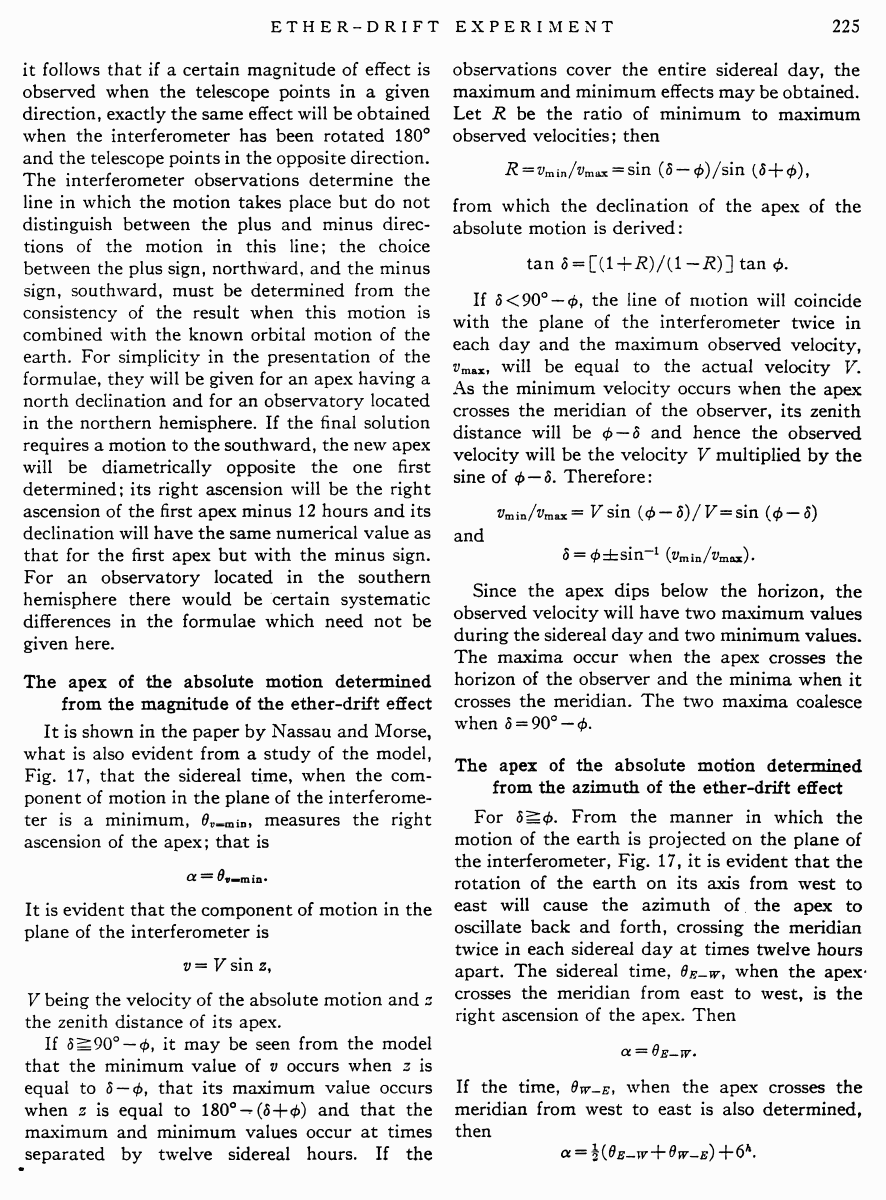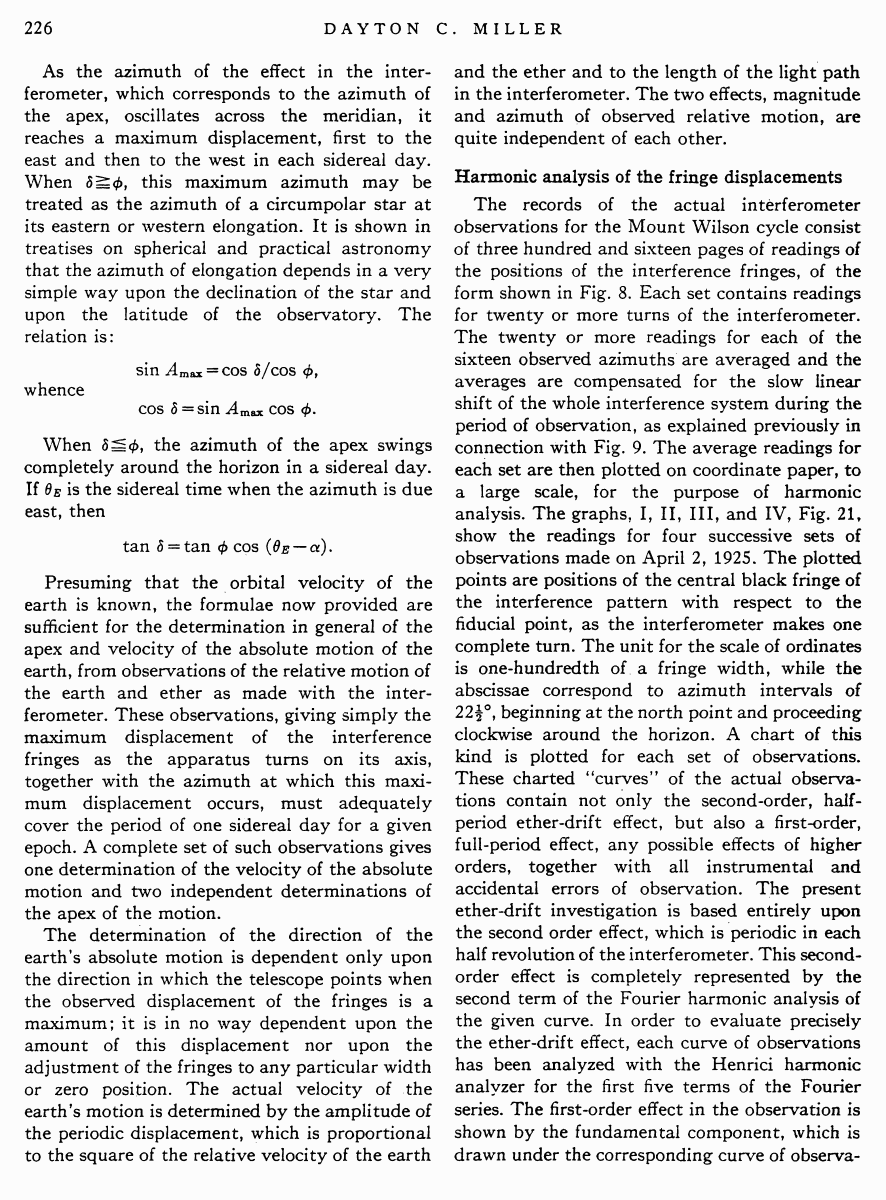# Miller D.C. The Ether-Drift Experiment and the Determination of the Absolute Motion of the Earth // Reviews of modern physics, Vol.5, July 1933

Start   PDF   <<<  Page 225   >>>203  204  205  206  207  208  209  210  211  212  213  214  215  216  217  218  219  220  221  222  223  224  225 226  227  228  229  230  231  232  233  234  235  236  237  238  239  240  241  242

it follows that if a certain magnitude of effect is observed when the telescope points in a given direction, exactly the same effect will be obtained when the interferometer has been rotated 180░ and the telescope points in the opposite direction. The interferometer observations determine the line in which the motion takes place but do not distinguish between the plus and minus directions of the motion in this line; the choice between the plus sign, northward, and the minus sign, southward, must be determined from the consistency of the result when this motion is combined with the known orbital motion of the earth. For simplicity in the presentation of the formulae, they will be given for an apex having a north declination and for an observatory located in the northern hemisphere. If the final solution requires a motion to the southward, the new apex will be diametrically opposite the one first determined; its right ascension will be the right ascension of the first apex minus 12 hours and its declination will have the same numerical value as that for the first apex but with the minus sign. For an observatory located in the southern hemisphere there would be certain systematic differences in the formulae which need not be given here.

The apex of the absolute motion determined from the magnitude of the ether-drift effect

It is shown in the paper by Nassau and Morse, what is also evident from a study of the model, Fig. 17, that the sidereal time, when the component of motion in the plane of the interferometer is a minimum, θv=min, measures the right ascension of the apex; that is

α = θv=min.

It is evident that the component of motion in the plane of the interferometer is

υ = V sin z,

V being the velocity of the absolute motion and z the zenith distance of its apex.

If δ ≥ 90░ ¢ φ, it may be seen from the model that the minimum value of v occurs when z is equal to δ ¢ φ, that its maximum value occurs when z is equal to 180░ ¢ (δ +φ) and that the maximum and minimum values occur at times separated by twelve sidereal hours. If the

observations cover the entire sidereal day, the maximum and minimum effects may be obtained. Let R be the ratio of minimum to maximum observed velocities; then

R = vmin / vmax = sin (δ ¢ φ) / sin (δ +φ),

from which the declination of the apex of the absolute motion is derived:

tan δ = [(1 +R) / (l ¢ R)] tan φ.

If δ < 90░ Ś φ, the line of motion will coincide with the plane of the interferometer twice in each day and the maximum observed velocity, vmax, will be equal to the actual velocity V. As the minimum velocity occurs when the apex crosses the meridian of the observer, its zenith distance will be φ ¢ δ and hence the observed velocity will be the velocity V multiplied by the sine of φ ¢ δ. Therefore:

vmin / vmax = V sin (φ ¢ δ) /V = sin (φ ¢ δ)

and

## δ = φ ▒ sin ¢1 (vmin/vmax).

Since the apex dips below the horizon, the observed velocity will have two maximum values during the sidereal day and two minimum values. The maxima occur when the apex crosses the horizon of the observer and the minima when it crosses the meridian. The two maxima coalesce when δ = 90░ ¢ φ.

The apex of the absolute motion determined from the azimuth of the ether-drift effect

For δ ≥ φ. From the manner in which the motion of the earth is projected on the plane of the interferometer, Fig. 17, it is evident that the rotation of the earth on its axis from west to east will cause the azimuth of the apex to oscillate back and forth, crossing the meridian twice in each sidereal day at times twelve hours apart. The sidereal time, θE¢W, when the apex crosses the meridian from east to west, is the right ascension of the apex. Then

α = θE¢W

If the time, θWŚE, when the apex crosses the meridian from west to east is also determined, then

α = (1/2)(θE¢W WŚE) +6h.

As the azimuth of the effect in the interferometer, which corresponds to the azimuth of the apex, oscillates across the meridian, it reaches a maximum displacement, first to the east and then to the west in each sidereal day. When δ ≥ φ, this maximum azimuth may be treated as the azimuth of a circumpolar star at its eastern or western elongation. It is shown in treatises on spherical and practical astronomy that the azimuth of elongation depends in a very simple way upon the declination of the star and upon the latitude of the observatory. The relation is:

sin Amax = cos δ /cos φ,

whence

cos δ = sin Amax cos φ.

When δ ≤ φ, the azimuth of the apex swings completely around the horizon in a sidereal day. If θE is the sidereal time when the azimuth is due east, then

tan δ = tan φ cos (θE ¢α).

Presuming that the orbital velocity of the earth is known, the formulae now provided are sufficient for the determination in general of the apex and velocity of the absolute motion of the earth, from observations of the relative motion of the earth and ether as made with the interferometer. These observations, giving simply the maximum displacement of the interference fringes as the apparatus turns on its axis, together with the azimuth at which this maximum displacement occurs, must adequately cover the period of one sidereal day for a given epoch. A complete set of such observations gives one determination of the velocity of the absolute motion and two independent determinations of the apex of the motion.

The determination of the direction of the earthÆs absolute motion is dependent only upon the direction in which the telescope points when the observed displacement of the fringes is a maximum; it is in no way dependent upon the amount of this displacement nor upon the adjustment of the fringes to any particular width or zero position. The actual velocity of the earthÆs motion is determined by the amplitude of the periodic displacement, which is proportional to the square of the relative velocity of the earth

and the ether and to the length of the light path in the interferometer. The two effects, magnitude and azimuth of observed relative motion, are quite independent of each other.

Harmonic analysis of the fringe displacements

The records of the actual interferometer observations for the Mount Wilson cycle consist of three hundred and sixteen pages of readings of the positions of the interference fringes, of the form shown in Fig. 8. Each set contains readings for twenty or more turns of the interferometer. The twenty or more readings for each of the sixteen observed azimuths are averaged and the averages are compensated for the slow linear shift of the whole interference system during the period of observation, as explained previously in connection with Fig. 9. The average readings for each set are then plotted on coordinate paper, to a large scale, for the purpose of harmonic analysis. The graphs, I, II, III, and IV, Fig. 21, show the readings for four successive sets of observations made on April 2, 1925. The plotted points are positions of the central black fringe of the interference pattern with respect to the fiducial point, as the interferometer makes one complete turn. The unit for the scale of ordinates is one-hundredth of a fringe width, while the abscissae correspond to azimuth intervals of 221/2░, beginning at the north point and proceeding clockwise around the horizon. A chart of this kind is plotted for each set of observations. These charted ōcurvesö of the actual observations contain not only the second-order, halfperiod ether-drift effect, but also a first-order, full-period effect, any possible effects of higher orders, together with all instrumental and accidental errors of observation. The present ether-drift investigation is based entirely upon the second order effect, which is periodic in each half revolution of the interferometer. This second-order effect is completely represented by the second term of the Fourier harmonic analysis of the given curve. In order to evaluate precisely the ether-drift effect, each curve of observations has been analyzed with the Henrici harmonic analyzer for the first five terms of the Fourier series. The first-order effect in the observation is shown by the fundamental component, which is drawn under the corresponding curve of observa